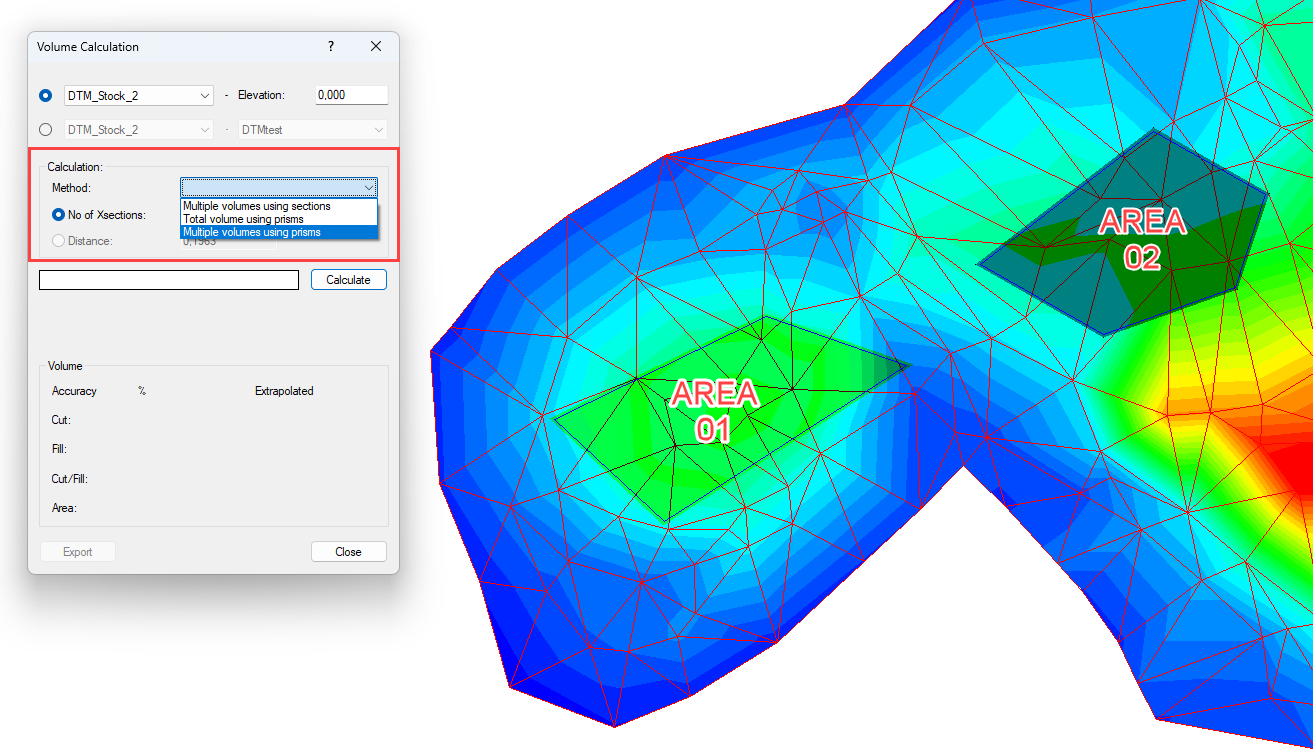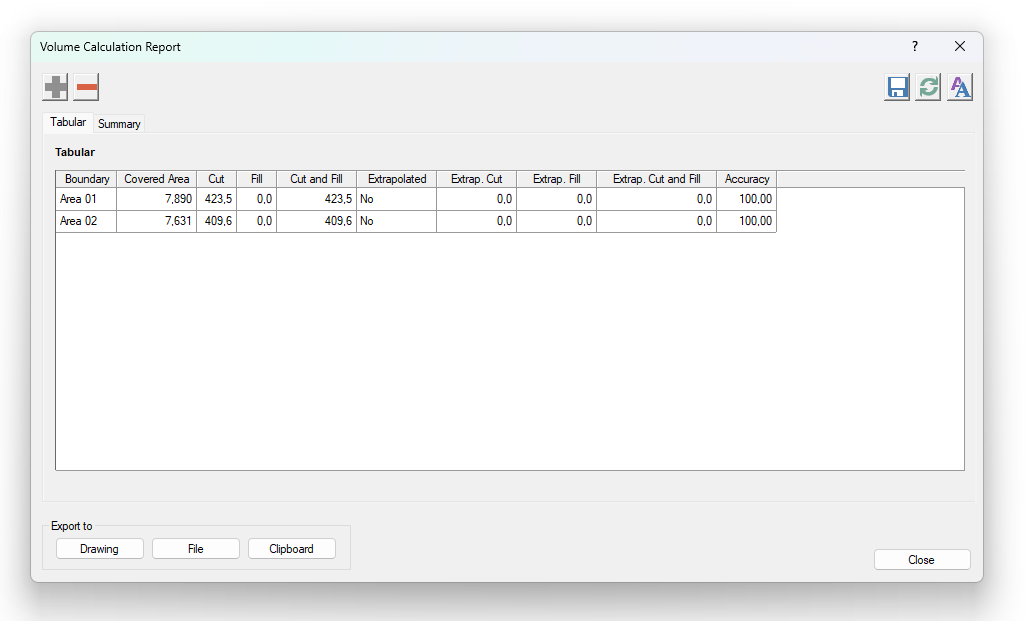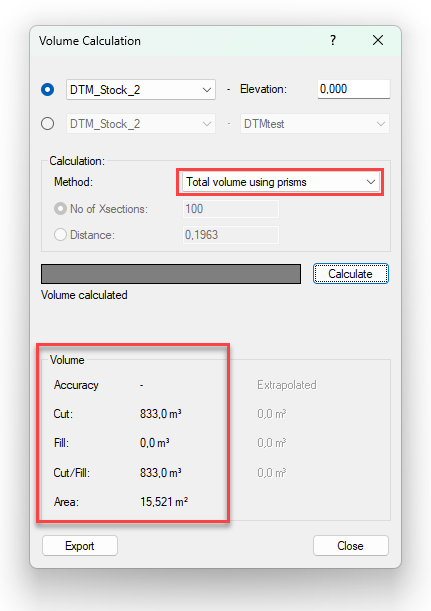Introduction

In earlier versions of Pythagoras, a boundary polygon could be used for a volume calculation on a Digital Terrain Model to determine the exact volume. From now on it will be possible to do the same with multiple boundary polygons at once!

This way, users will gain time and don’t need to set up different calculations manually.

To go even further, we've added a different way of calculating these volumes to get an even more accurate result: The prismoidal method. If you use this method there's no more need to work with Xsections, because the volume calculation is based on triangular prisms.If you calculate, based on multiple polygons using prisms, a new tab ‘Tabular’ will be generated in the Volume Calculation Report. Here you’ll get an overview of the different boundaries with the calculated data. This of course can be edited and exported into the drawing or to an external file.You can also directly calculate the total volume of both areas by choosing 'Total volume using prisms':Video